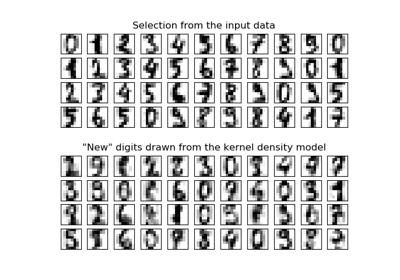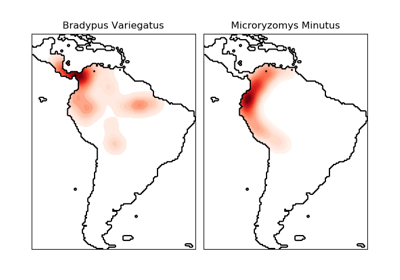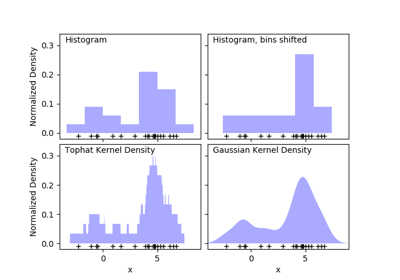/scikit-learn

# sklearn.neighbors.KernelDensity

`class sklearn.neighbors.KernelDensity(bandwidth=1.0, algorithm=’auto’, kernel=’gaussian’, metric=’euclidean’, atol=0, rtol=0, breadth_first=True, leaf_size=40, metric_params=None)` [source]

Kernel Density Estimation

Read more in the User Guide.

Parameters: `bandwidth : float` The bandwidth of the kernel. `algorithm : string` The tree algorithm to use. Valid options are [‘kd_tree’|’ball_tree’|’auto’]. Default is ‘auto’. `kernel : string` The kernel to use. Valid kernels are [‘gaussian’|’tophat’|’epanechnikov’|’exponential’|’linear’|’cosine’] Default is ‘gaussian’. `metric : string` The distance metric to use. Note that not all metrics are valid with all algorithms. Refer to the documentation of `BallTree` and `KDTree` for a description of available algorithms. Note that the normalization of the density output is correct only for the Euclidean distance metric. Default is ‘euclidean’. `atol : float` The desired absolute tolerance of the result. A larger tolerance will generally lead to faster execution. Default is 0. `rtol : float` The desired relative tolerance of the result. A larger tolerance will generally lead to faster execution. Default is 1E-8. `breadth_first : boolean` If true (default), use a breadth-first approach to the problem. Otherwise use a depth-first approach. `leaf_size : int` Specify the leaf size of the underlying tree. See `BallTree` or `KDTree` for details. Default is 40. `metric_params : dict` Additional parameters to be passed to the tree for use with the metric. For more information, see the documentation of `BallTree` or `KDTree`.

#### Methods

 `fit`(X[, y, sample_weight]) Fit the Kernel Density model on the data. `get_params`([deep]) Get parameters for this estimator. `sample`([n_samples, random_state]) Generate random samples from the model. `score`(X[, y]) Compute the total log probability under the model. `score_samples`(X) Evaluate the density model on the data. `set_params`(**params) Set the parameters of this estimator.
`__init__(bandwidth=1.0, algorithm=’auto’, kernel=’gaussian’, metric=’euclidean’, atol=0, rtol=0, breadth_first=True, leaf_size=40, metric_params=None)` [source]
`fit(X, y=None, sample_weight=None)` [source]

Fit the Kernel Density model on the data.

Parameters: `X : array_like, shape (n_samples, n_features)` List of n_features-dimensional data points. Each row corresponds to a single data point. `sample_weight : array_like, shape (n_samples,), optional` List of sample weights attached to the data X.
`get_params(deep=True)` [source]

Get parameters for this estimator.

Parameters: `deep : boolean, optional` If True, will return the parameters for this estimator and contained subobjects that are estimators. `params : mapping of string to any` Parameter names mapped to their values.
`sample(n_samples=1, random_state=None)` [source]

Generate random samples from the model.

Currently, this is implemented only for gaussian and tophat kernels.

Parameters: `n_samples : int, optional` Number of samples to generate. Defaults to 1. `random_state : int, RandomState instance or None. default to None` If int, random_state is the seed used by the random number generator; If RandomState instance, random_state is the random number generator; If None, the random number generator is the RandomState instance used by `np.random`. `X : array_like, shape (n_samples, n_features)` List of samples.
`score(X, y=None)` [source]

Compute the total log probability under the model.

Parameters: `X : array_like, shape (n_samples, n_features)` List of n_features-dimensional data points. Each row corresponds to a single data point. `logprob : float` Total log-likelihood of the data in X.
`score_samples(X)` [source]

Evaluate the density model on the data.

Parameters: `X : array_like, shape (n_samples, n_features)` An array of points to query. Last dimension should match dimension of training data (n_features). `density : ndarray, shape (n_samples,)` The array of log(density) evaluations.
`set_params(**params)` [source]

Set the parameters of this estimator.

The method works on simple estimators as well as on nested objects (such as pipelines). The latter have parameters of the form `<component>__<parameter>` so that it’s possible to update each component of a nested object.

Returns: self

## Examples using `sklearn.neighbors.KernelDensity`Kernel Density EstimationKernel Density Estimate of Species DistributionsSimple 1D Kernel Density Estimation

© 2007–2018 The scikit-learn developers
Licensed under the 3-clause BSD License.
http://scikit-learn.org/stable/modules/generated/sklearn.neighbors.KernelDensity.html